# CBSE Sample Paper for Class 10 Maths Basic 2020-21

CBSE has released official sample paper for Class  Maths Basic 2020-21 which will be useful knowing the latest pattern and types of questions that can come in the examinations. The questions has been asked from variety of topics so that you can prepare well for the examinations.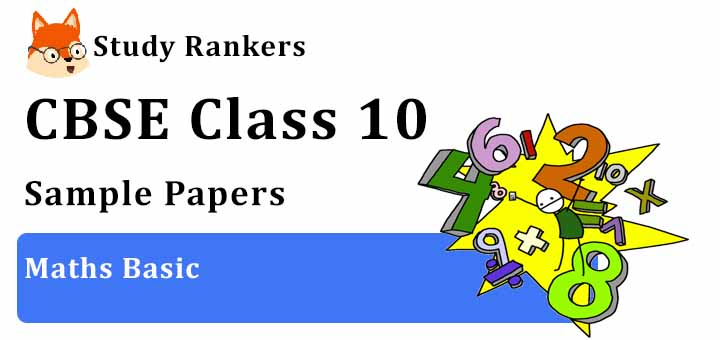General Instructions:
1. This question paper contains two parts A and B.
2. Both Part A and Part B have internal choices.

Part – A:
1. It consists of two sections- I and II
2. Section I has 16 questions. Internal choice is provided in 5 questions.
3. Section II has four case study-based questions. Each case study has 5 case-based sub-parts.
An examinee is to attempt any 4 out of 5 sub-parts.

Part – B:
1. Question No 21 to 26 are Very short answer Type questions of 2 mark each,
2. Question No 27 to 33 are Short Answer Type questions of 3 marks each
3. Question No 34 to 36 are Long Answer Type questions of 5 marks each.
4. Internal choice is provided in 2 questions of 2 marks, 2 questions of 3 marks and 1 question of 5
marks.

Part A

Section-I

1. Express 156 as the product of primes. (1)

2. Write a quadratic polynomial, sum of whose zeroes is 2 and product is -8. (1)

3. Given that HCF (96,404) is 4, find the LCM ( 96,404).
OR
State the fundamental Theorem of Arithmetic. (1)

4. On comparing the ratios of the coefficients, find out whether the pair of equations x – 2y =0 and 3x + 4y -20 =0 is consistent or inconsistent. (1)

5. If a and b are co-prime numbers, then find the HCF (a, b). (1)

6. Find the area of a sector of a circle with radius 6cm if angle of the sector is 60°. (Take 𝜋 = 22/7)
OR
A horse tied to a pole with 28m long rope. Find the perimeter of the field where the horse can graze. (Take 𝜋 = 22/7) (1)

7. In the given fig. DE || BC, ∠ADE =70° and ∠BAC=50°, then angle ∠BCA = ______OR
In the given figure, AD = 2cm, BD = 3 cm, AE = 3.5 cm and AC = 7 cm. Is DE parallel to BC? (1)8. The cost of fencing a circular field at the rate of Rs.24 per metre is Rs. 5280. Find the radius of the field. (1)

9. A tree breaks due to storm and the broken part bends so that the top of the tree touches the ground where it makes an angle 30° . The distance between the foot of the tree to the point where the top touches the ground is 8m. Find the height of the tree from where it is broken. (1)

10. If the perimeter and the area of a circle are numerically equal, then find the radius of the circle. (1)

11. Write the empirical relationship among mean, median and mode. (1)

12. To divide a line segment BC internally in the ratio 3 : 5, we draw a ray BX such that ∠CBX is an acute angle. What will be the minimum number of points to be located at equal distances, on ray BX? (1)

13. For what values of p does the pair of equations 4x + p y +8 =0 and 2x +2y +2 =0 has unique solution?
OR
What type of straight lines will be represented by the system of equations 2x + 3y =5 and 4x + 6y = 7? (1)

14. A bag contains 3 red balls and 5 black balls. A ball is drawn at random from the bag. What is the probability that the ball drawn is red?
OR
A die is thrown once. What is the probability of getting a prime number? (1)

15. A tower stands vertically on the ground. From a point on the ground, which is 15m away from the foot of the tower, the angle of elevation of the top of the tower is found to be 60°.Find the height of the tower. (1)

16. Probability of an event E + Probability of the event E̅ ( not E) is,_______ (1)

Section-II

Case study-based questions are compulsory. Attempt any 4 sub parts from each question. Each question carries 1 mark.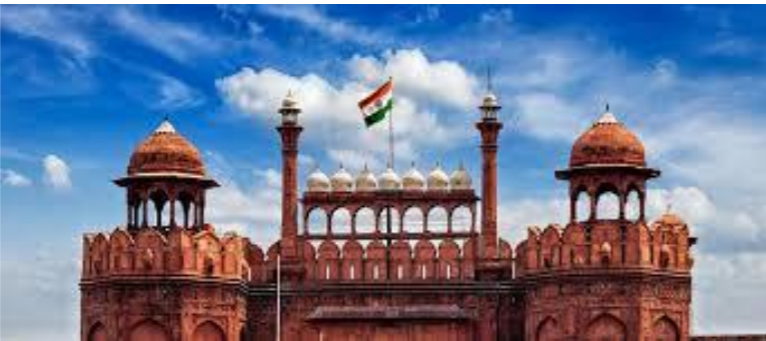Mathematics teacher of a school took her 10th standard students to show Red fort. It was a part of their Educational trip. The teacher had interest in history as well. She narrated the facts of Red fort to students. Then the teacher said in this monument one can find combination of solid figures. There are 2 pillars which are cylindrical in shape. Also 2 domes at the corners which are hemispherical.7 smaller domes at the centre. Flag hoisting ceremony on Independence Day takes place near these domes.

i) How much cloth material will be required to cover 2 big domes each of radius 2.5 metres? (Take 𝜋 = 22/7)
a) 75m2
b) 78.57m2
c) 87.47m2
d) 25.8m2

ii) Write the formula to find the volume of a cylindrical pillar.
a) πr2h
b) πrl
c) πr(l + r)
d) 2πr

iii) Find the lateral surface area of two pillars if height of the pillar is 7m and radius of the base is 1.4m.
a) 112.3cm2
b) 123.2m2
c) 90m2
d) 345.2cm2

iv) How much is the volume of a hemisphere if the radius of the base is 3.5m?
a) 85.9 m3
b) 80 m3
c) 98 m3
d) 89.83 m3

v) What is the ratio of sum of volumes of two hemispheres of radius 1cm each to the volume of a sphere of radius 2 cm?
a) 1:1
b) 1:8
c) 8 :1
d) 1:16

18. Class X students of a secondary school in Krishnagar have been allotted a rectangular plot of a land for gardening activity. Saplings of Gulmohar are planted on the boundary at a distance of 1m from each other. There is a triangular grassy lawn in the plot as shown in the fig. The students are to sow seeds of flowering plants on the remaining area of the plot. (1×5=5)Considering A as origin, answer question (i) to (v)

i) Considering A as the origin, what are the coordinates of A?
a) (0,1)
b) (1,0)
c) (0,0)
d)(-1,-1)

ii) What are the coordinates of P?
a) (4,6)
b)( 6,4)
c) (4,5)
d) (5,4)

iii) What are the coordinates of R?
a) (6,5)
b) (5,6)
c) ( 6,0)
d) (7,4)

iv) What are the coordinates of D?
a) (16,0)
b) (0,0)
c) (0,16)
d) (16,1)

v) What are the coordinate of P if D is taken as the origin?
a) ( 12,2)
b ) (-12,6)
c) (12,3)
d) (6,10)

19.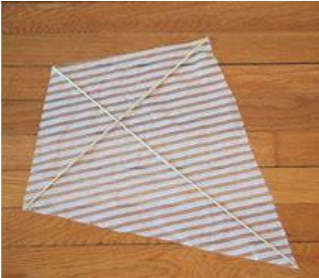Rahul is studying in X Standard. He is making a kite to fly it on a Sunday. Few questions came to his mind while making the kite. Give answers to his questions by looking at the figure. (1×5=5)

i) Rahul tied the sticks at what angles to each other?
a) 30°
b) 60°
c) 90°
d) 60°

ii) Which is the correct similarity criteria applicable for smaller triangles at the upper part of this kite?
a) RHS
b) SAS
c) SSA
d) AAS

iii) Sides of two similar triangles are in the ratio 4:9. Corresponding medians of these triangles are in the ratio,
a) 2:3
b) 4:9
c) 81:16
d) 16:81

iv) In a triangle, if square of one side is equal to the sum of the squares of the other two sides, then the angle opposite the first side is a right angle. This theorem is called as,
a) Pythagoras theorem
b) Thales theorem
c) Converse of Thales theorem
d) Converse of Pythagoras theorem

v) What is the area of the kite, formed by two perpendicular sticks of length 6 cm and 8 cm?
a) 48 cm2
b) 14 cm2
c) 24 cm2
d) 96 cm2

20. Due to heavy storm an electric wire got bent as shown in the figure. It followed a mathematical shape. Answer the following questions below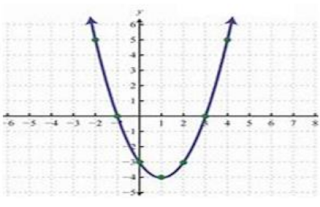i) Name the shape in which the wire is bent
a) Spiral
b) ellipse
c) linear
d) Parabola

ii) How many zeroes are there for the polynomial (shape of the wire)
a) 2
b) 3
c) 1
d) 0

iii) The zeroes of the polynomial are
a) -1, 5
b) -1, 3
c) 3, 5
d) -4, 2

iv) What will be the expression of the polynomial?
a) x2+2x -3
b) x2 -2x +3
c) x2 - 2x -3
d) x2 +2x+3

v) What is the value of the polynomial if x = -1?
a) 6
b) -18
c)) 18
d) 0

Part –B

All questions are compulsory. In case of internal choices, attempt anyone.

21. Find the coordinates of the point which divides the line segment joining the points (4, -3) and (8,5) in the ratio 3:1 internally. (2)
OR
Find a relation between x and y such that the point (x,y) is equidistant from the points (7,1) and (3,5).

22.

In the fig. if LM II CB and LN II CD, prove that AM/MB = AN/ND

23. A quadrilateral ABCD is drawn to circumscribe a circle. Prove that AB + CD = AD + BC.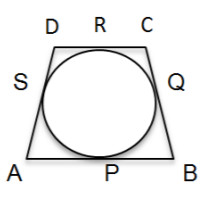24. Draw a line segment of length 7.8 cm and divide it in the ratio 5:8. Measure the two parts.

25. 25 Given 15 cot A =8, find sin A and sec A.
OR
Find tan P – cot R26. How many terms of the A. P : 9,17,25, .......must be taken to give a sum 636? (2)

Part –B

All questions are compulsory. In case of internal choices, attempt anyone.

27. Prove that √3 is an irrational number. (3)

28. Two tangents TP and TQ are drawn to a circle with centre O from an external point T. Prove that ∠PTQ = 2∠OPQ. (3)29. 29 Meena went to a bank to withdraw Rs.2,000. She asked the cashier to give her Rs.50 and Rs.100 notes only. Meena got 25 notes in all. Find how many notes of Rs.50 and Rs.100 she received. (3)

30 A box contains 90 discs which are numbered from 1 to 90. If one disc is drawn at random from the box, find the probability that it bears (3)
(i) a two-digit number
(ii) a perfect square number.
(iii) a number divisible by 5.
OR
One card is drawn from a well shuffled deck of 52 cards. Find the probability of getting
(i) A king of red colour.
(iii) The queen of diamonds

31. Metallic spheres of radii 6cm, 8cm and 10cm respectively are melted to form a solid sphere. Find the radius of the resulting sphere. (3)

32. Prove that33. A motor boat whose speed in still water is 18 km/h, takes 1 hour more to go 24 km upstream than to return downstream to the same spot. Find the speed of the stream.
OR
Find two consecutive odd positive integers, sum of whose squares is 290. (3)

Part –B

All questions are compulsory. In case of internal choices, attempt anyone.

34. The angles of depression of the top and bottom of a 8m tall building from the top of a multi storied building are 30° and 45°, respectively. Find the height of the multi storied building and the distance between the two buildings. (5)
OR
A 1.2m tall girl spots a balloon moving with the wind in a horizontal line at a height 88.2 m from the ground. The angle of elevation of the balloon from the eyes of the girl at any instant is 60°.After sometime, the angle of elevation reduces 30°.Find the distance travelled by the balloon during the interval.35. The pth, qth and rth terms of an A.P. are a, b and c respectively. (5)
Show that a(q – r) + b(r-p) + c(p – q) = 0

36. A survey regarding the heights in (cm) of 51 girls of class X of a school was conducted and the following data was obtained. Find the median height and the mean using the formulae. (5)